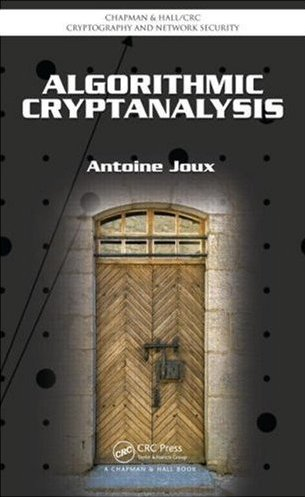### Algorithmic Cryptanalysis

• Libraries & Programs
• IACR
• Cryptographer's world
• CRC Press
• Book on CRC Press

# Hints for Exercises (Chapter 12)

• Exercise 1: Xoring the two encryption deletes the one-time key.
• Exercise 3: Instead of using a function from t bits to 1, we now have a function from t+k bits to k+1.
• Exercise 5: At a given point in time, the possible output values from A and B are either equal or different. If they are equal, predicting a bit of A is easy. What happens when they are different.
• Exercise 7: For plain parity checks, you can always assume that the first output bit is included.
• Exercise 9: The new bit is a function of the stored bits.

Credits for page styles: Dynamic Drive CSS Library